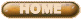ANDRES J. WASHINGTON'S RESEARCH

Here is an interesting article by a Fingerprint expert. He  has some fascinating applications to the Great Pyramid.  At the bottom of this page, click on Part 2 to see some of his research and information on fingerprints

Fingerprint Geometric Analysis

Time Times Time = Time (D² · Y = Z) I have often wondered , how much time would exist if time was to be multiplied by time. So, I selected two units of time; the day and the year. I also selected a common denominator, the hour.

The number of hours in a day, 24 multiplied by the number of hours in a year, 8,760 (Note that 365 x 24 = 8,760) equals 210,240 hours.

(24(365 x 24) = 210,240 or 24²(365) = 210,240 or 24 x 8,760 = 210,240)

To determine the number of days which exist in 210,240 hours divide 210,240 by 24; 210,240 ÷ 24 =8,760 days. (Note here that 8,760 represents days and not hours as before.) To determine the number of years which exist in 8,760 days divide 8,760 by 365; 8,760 ÷ 365 = 24 years.

In conclusion, the number of hours in a day, 24 multiplied by the number of hours in a year, 8,760 equals 210,240 hours which is equivalent to 24 years of time. The interesting aspect about this is that the number 24 is coincidental to hours and years.